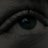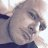# How do you make a timeframe a variable?##### Well-known member
In some code I'm working on I only want the condition to apply if it happens after hours. I tried this and it didn't work,
def Start = 1600;
def End = 930;
def conf = SecondsFromTime(Start) >= 0 and SecondsFromTime(End) <= 0;

When I tried to use conf as a variable it didn't work and the condition was still applied throughout the whole day even though adding conf as a condition should have only let it signal ah.
Any ideas how this is done? This could be useful for many things.#### Prison Mike

##### Member
This may or may not help but I used this to find overnight ATR. Maybe it can be chopped up.

Code:
``````input n = 10;
def bar = BarNumber();
def nan = Double.NaN;
input Agg = AggregationPeriod.day;
input Length = 500;
input AverageType = AverageType.SIMPLE;
input ATRMulti = .75;
def h = high(period = Agg);
def l = low(period = Agg);
def c = close(period = Agg);

def PMhrs = RegularTradingStart (GetYYYYMMDD()) > GetTime();
def RMhrs = RegularTradingStart (GetYYYYMMDD()) < GetTime();
def PMStart = RMhrs and PMhrs;
def PMclose = CompoundValue(1, if PMStart then h else if PMhrs then Max(h, PMclose) else PMclose, 0);
def PMHigh = CompoundValue(1, if PMStart then h else if PMhrs then Max(h, PMHigh) else PMHigh, 0);
def PMLow = CompoundValue(1, if PMStart then l else if PMhrs then Min(l, PMLow) else PMLow, 0);
def highBar = if PMhrs and h == PMHigh then bar else nan;
def lowBar = if PMhrs and l == PMLow then bar else nan;
def PMHighBar = if bar == HighestAll(highBar) then PMHigh else PMHighBar;
def PMLowBar = if bar == HighestAll(lowBar) then PMLow else PMLowBar;

def ONATR = MovingAverage(AverageType, TrueRange(h, c, l), Length) * ATRMulti;``````

•QQE MTF (Multi Timeframe) for ThinkorSwim Indicators 11How do I use multi timeframe in a watchlist column or scan? Questions 2